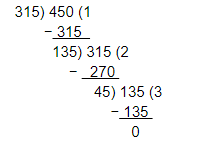# Find the largest number which divides 320 and 457 leaving remainders 5 and 7 respectively.

Question:

Find the largest number which divides 320 and 457 leaving remainders 5 and 7 respectively.

Solution:

We know that the required number divides 315 (320 − 5) and 450 (457 − 7).
∴ Required number = HCF (315, 450)
On applying Euclid's lemma, we get:Therefore, the HCF of 315 and 450 is 45.
Hence, the required number is 45.